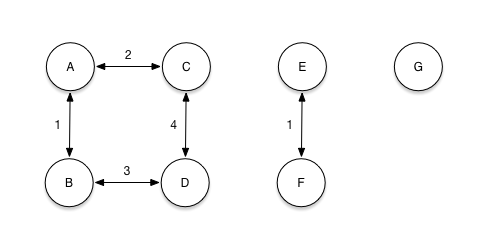Package

# purescript-digraph

Repository
nullobject/purescript-digraph
MIT
nullobject
Published on
2019-01-12T07:09:10ZA directed graph library for PureScript.

## ExamplesThe directed graph pictured above can be represented with an `AdjacencyList`. An adjacency list is a list of tuples that contain a vertex and a list of edges to its adjacent vertices. A `Graph` can be constructed from an `AdjacencyList`.

```let edges = fromFoldable \$
[ Tuple 'A' (fromFoldable [Tuple 'B' 1, Tuple 'C' 2])
, Tuple 'B' (fromFoldable [Tuple 'A' 1, Tuple 'D' 3])
, Tuple 'C' (fromFoldable [Tuple 'A' 2, Tuple 'D' 4])
, Tuple 'D' (fromFoldable [Tuple 'B' 3, Tuple 'C' 4])
, Tuple 'E' (fromFoldable [Tuple 'F' 1])
, Tuple 'F' (fromFoldable [Tuple 'E' 1])
, Tuple 'G' (fromFoldable [])
]

### Size

The `size` function returns the number of vertices in a graph.

```size graph
-- 7```

### Vertices

The `vertices` function returns a list of the vertices in a graph.

```vertices graph
-- ['A', 'B', 'C', 'D', 'E', 'F', 'G']```

### Edges

The `isAdjacent` function returns true if two vertices are connected by an edge.

```isAdjacent 'A' 'B' graph
-- true```

The `weight` function returns the weight of the edge between two vertices.

```weight 'A' 'B' graph
-- Just 1```

The `adjacent` function returns all vertices connected to a vertex by an edge. The `adjacent'` function will also include the weight of the edges.

```adjacent 'A' graph
-- ['B', 'C']

-- [Tuple 'B' 1, Tuple 'C' 2]```

### Shortest path

The `shortestPath` function calculates the shortest path between two vertices using Dijkstra'a algorithm.

```shortestPath 'A' 'D' graph
-- ['A', 'B', 'D']```

### Connected components

The `connectedComponents` function calculates a list of connected components in a graph.

A connected component is a maximal subgraph in which any two vertices are connected to each other by a path.

```connectedComponents graph
-- [Graph, Graph, Graph]```

### Building

An empty graph can be constructed and updated.

```(insertEdge 'A' 'B' 1 <<< insertVertex 'A' <<< insertVertex 'B') empty
-- Graph```

Data.Graph

Modules
Data.Graph
Dependencies